# C博客作业05--指针

| 这个作业属于哪个班级 | C语言--网络2011/2012 |
| ---- | ---- | ---- |
| 这个作业的地址 | C博客作业05--指针 |
| 这个作业的目标 | 学习指针相关内容 |
| 姓名 | 曹卉潼 |

# 0.展示PTA总分（0----2）# 1.本章学习总结（3分）

## 1.1 指针定义、指针相关运算、指针做函数参数。

### 指针定义：

类型名  *指针变量名


### 指针的基本运算

• 1.取地址运算和间接访问运算
单目运算符&用于给出变量的地址

eg:
   int *p,a=3;
p=&a;


• 2.赋值运算
一旦指针贝定月赋值后，就可以如同其它类型变量一样进行赋值运算。
eg:
   int a=3,*p1,*p2;
p1=&a;
p2=p1;


• 3.指针变量的初始化
不能用数值作为指针变量的初值，可以初始化为空指针

eg:
   int *p=0;
int *p=NULL；


### 指针做函数参数：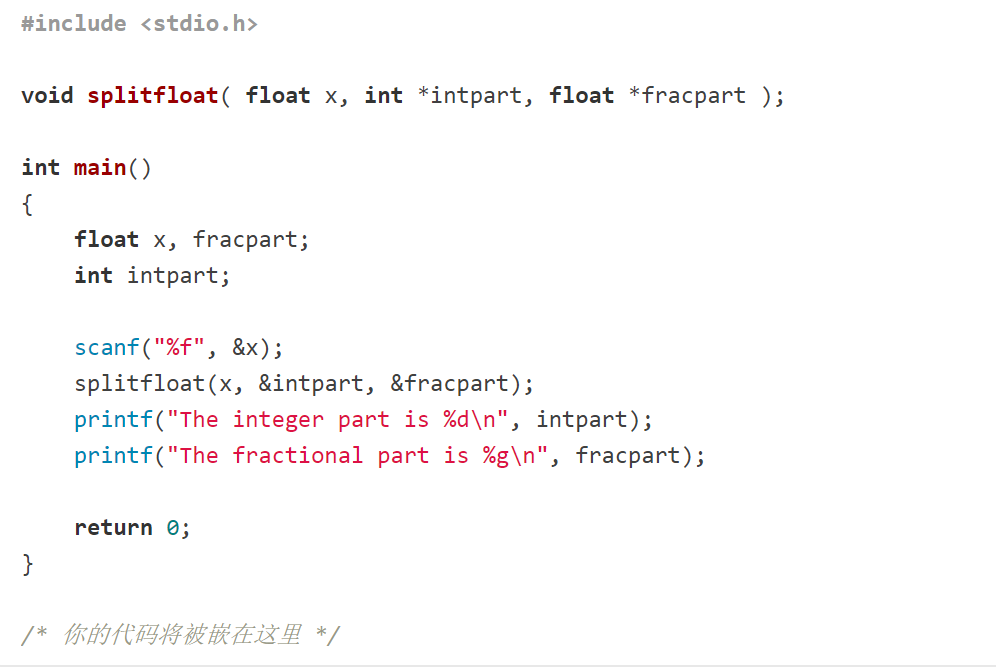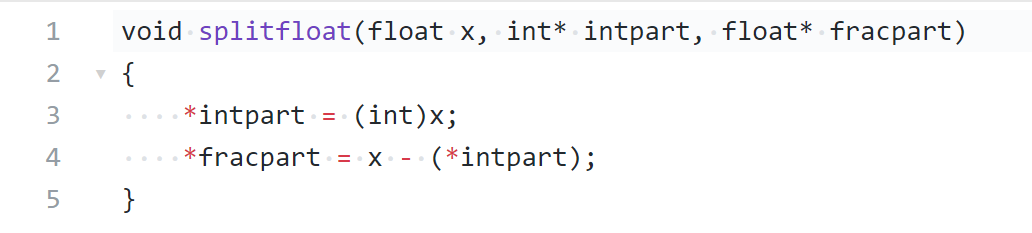• 5.指针自增运算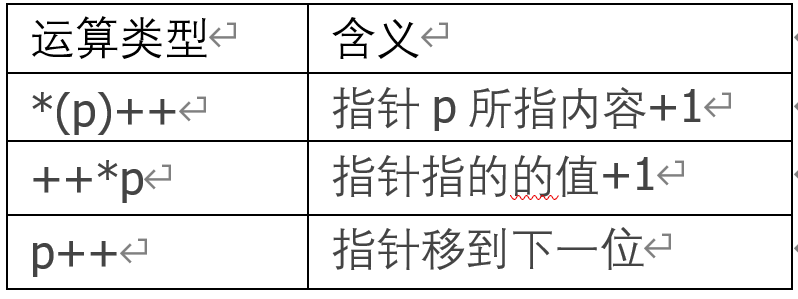## 1.2 字符指针

char *p = "i am 006";//将保存在常量存储区的"i am 006"的首地址赋值给ptr

char *p;
p = "i am 006";//是等价的，注意不能理解为将字符串赋值给ptr


• 1.字符串的输入和输出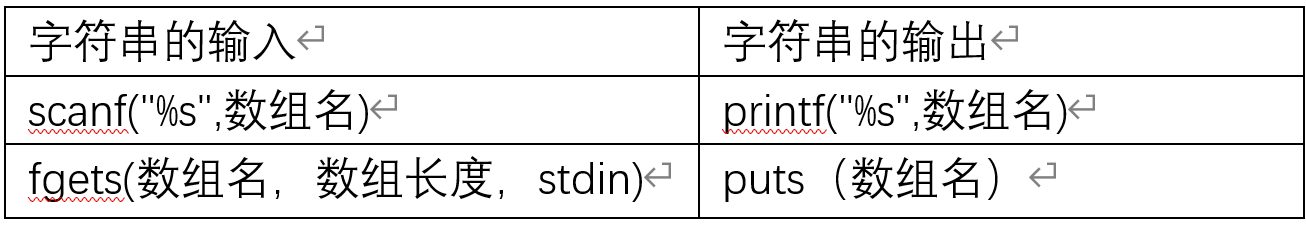• 2.字符串复制函数

 char *strcpy(char( *s1,char *s2)或
<font color="#0000dd">strcpy(s1,s2);</font><br />


s1必须是字符型数组基地址，参数s2可以使字符数组名或字符串常量

eg:

 int i;
char s1,s2,s="CHINA";
strcpy(str1,s);
strcpy(str2,"abc");

• 3.字符串连接函数
strcat(s1,s2);


eg:

char s1="hello",s2="world";
strcat(s1,s2);

• 4.字符串比较函数
  strcmp(s1,s2);


eg:

strcmp(s1,s2)>0；//返回一个正数
strcmp(s1,s2)>0；//返回一个负数
strcmp(s1,s2)==0；//返回0

• 5.计算字符串长度函数
 strlen(s1,s2);//'\0'不计入所计算出的长度

• 6.free函数
free（）//释放动态内存空间

• 拓展字符串函数
(1)
  strpbrk(str1,str2)
char *strpbrk(const char *str1, const char *str2)
//检索字符串 str1 中第一个匹配字符串str2 中字符的字符


(2)

strstr()
char *strstr(const char *haystack, const char *needle)
//字符串 haystack 中查找第一次出现字符串 needle 的位置


(3)

islower()：//如果 c 有相对应的小写字母，则该函数返回 c 的小写字母，



(4)

toupper()：如果 c 有相对应的大写字母，则该函数返回 c 的大写字母，否则 c 保持不变。


char* s1;
char* s2;
s1 = (char*)malloc(1200 * sizeof(char));//申请1200个char单位的空间;
s2 = (char*)malloc(3600 * sizeof(char));//申请3600个char单位的空间;
free(s1);
free(s2);


## 1.3 指针做函数返回值

C语言允许函数的返回值是一个指针（地址），我们将这样的函数称为指针函数。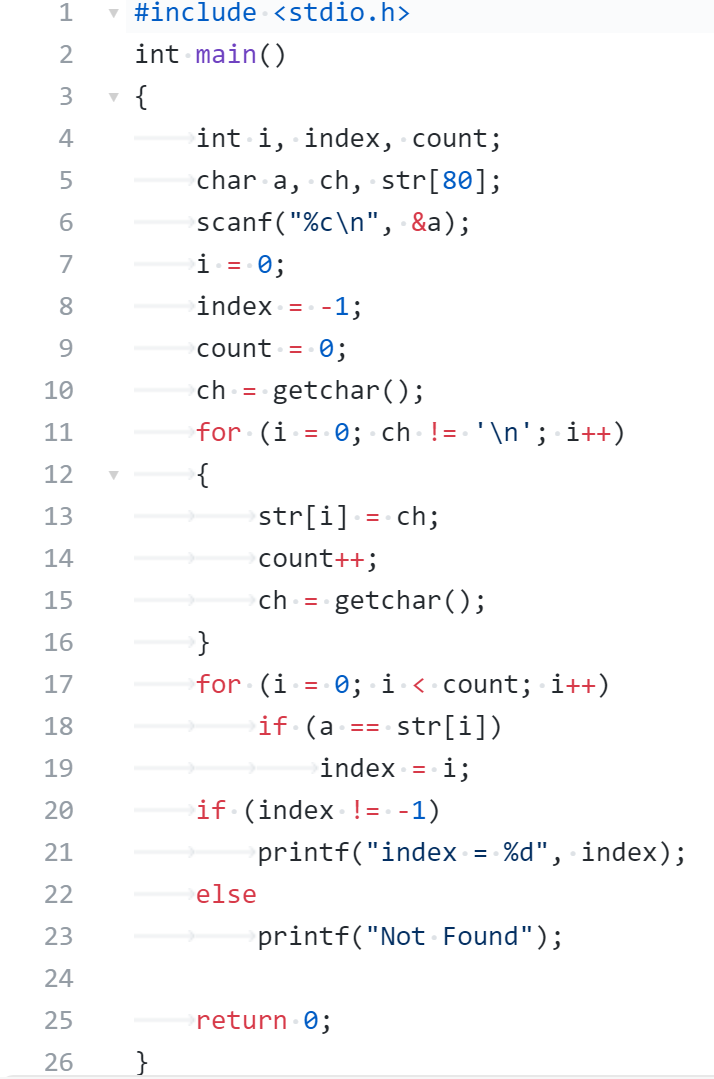## 1.4 动态内存分配

1)申请方式： 栈区内存由系统自动分配，函数结束时释放；堆区内存由程序员自己申请，并指明大小，用户忘释放时，会造成内存泄露，不过进程结束时会由系统回收。
2)申请后系统的响应： 只要栈的剩余空间大于所申请的空间，系统将为程序提供内存，否则将报异常提示栈溢出；堆区，空闲链表，分配与回收机制，会产生碎片问题（外部碎片）-->（固定分区存在内部碎片（分配大于实际），可变分区存在外部碎片（太碎无法分配））。
3)申请大小的限制：栈是1或者2M，可以自己改，但是最大不超过8M；堆，看主机是多少位的，如果是32位，就是4G
4)申请效率：栈由系统自动分配，速度较快，程序员无法控制；堆是由new分配的内存，一般速度较慢，而且容易导致内存碎片，但是用起来方便！
5)存储内容：栈，函数调用（返回值，各个参数，局部变量（静态变量不入栈））；堆，一般在堆的头部用一个字节存放堆的大小，堆中的具体内容由程序员安排。
6)存取效率的比较：栈比堆快。
7)管理方式不同：栈，数据结构中的栈；堆，链表
8)生长方向：栈，高到低；堆，低到高

malloc动态申请内存

int n;//记录输入总个数
int *p;
scanf("%d",&n);
p=(int *)malloc(n * (sizeof(int));


calloc动态申请内存

int n;//记录输入总个数
int *p;
scanf("%d",&n);
p=(int *)calloc(n , (sizeof(int));


realloc动态申请内存

int n;//记录输入总个数
int **p=NULL;
scanf("%d",&n);
p=(int **)calloc(p , n * (sizeof(int *));


free（）；


## 1.6 二级指针

int i;
char *color[]={"red","blue","yellow","green","black"};
char **pc;
for(i=0;i<5;i++)
printf("%s",*(pc+i));


## 1.7 行指针、列指针

• 行指针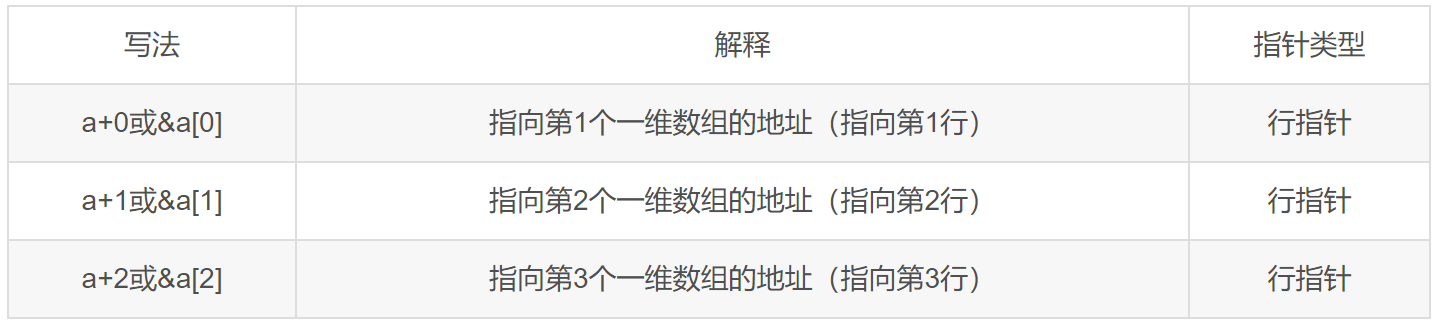• 列指针# 2.PTA实验作业（7分）

## 2.1 删除字符串中的子串（2分）

### 2.1.1 伪代码

输入主串;

for i=0 to len1 do i++
k=i;//储存当前主串所在位置
int j=0
while  主串元素与子串相同且该元素后都不是最后一个时
k++;j++
end while
if  str2[j] == '\0' ||str2[j] == '\n'
for int m=0 to len2 do m++
for int t=i to len1 do t++
将数组str1[]往前移,达到删除子串的目的
end for
给数组最后一个字符赋0
end for
len1=len1-len2
i=-1
end if
end for



### 2.1.2 代码截图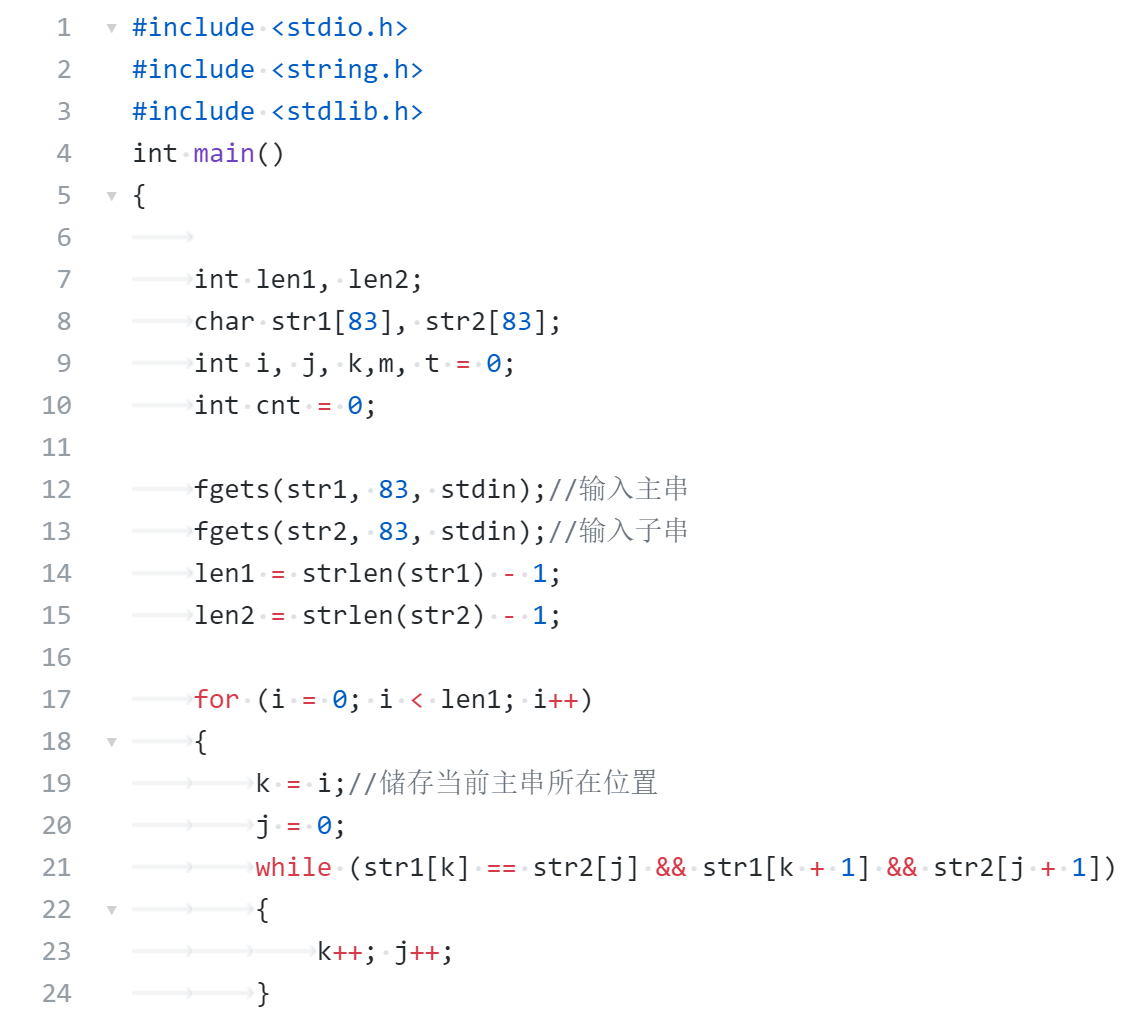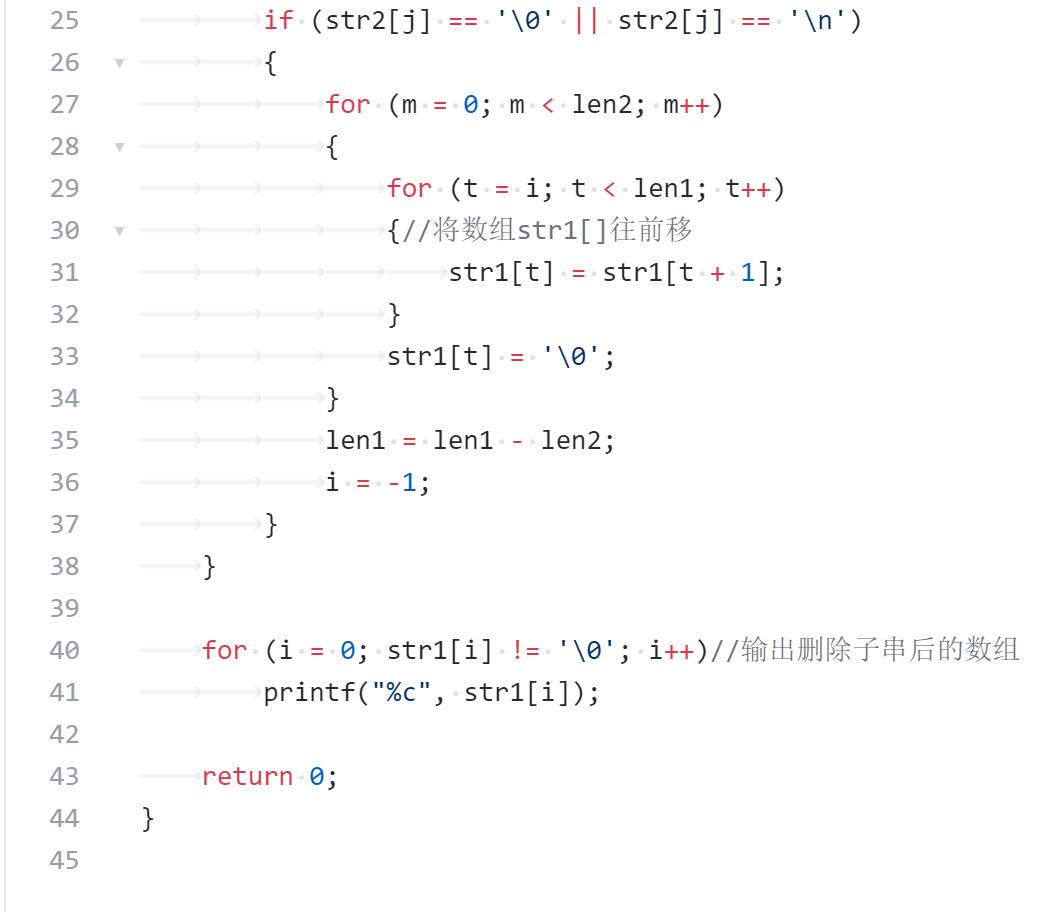### 2.1.3 找一份同学代码（尽量找思路和自己差距较大同学代码）比较，说明各自代码特点。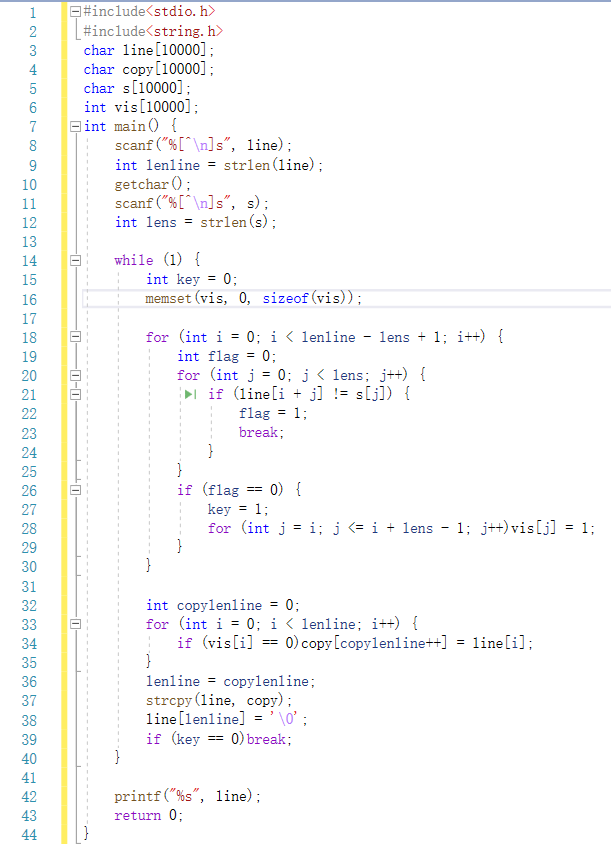## 2.2 合并2个有序数组（2分)

### 2.2.1 伪代码

定义一个整型指针*c;

for int k=0 to  do k++
if  a[i=0]<b[j=0]
把a[i]储存在c[k]中
i++
else
把b[j]储存在c[k]中
j++;
end if
end for
while  i<m
依次把a数组中剩余的元素给c
end while
while  j<n
依次把b数组中剩余的元素给c
end while
for i=0 to m+n do i++
把c中的元素存入a数组



### 2.2.2 代码截图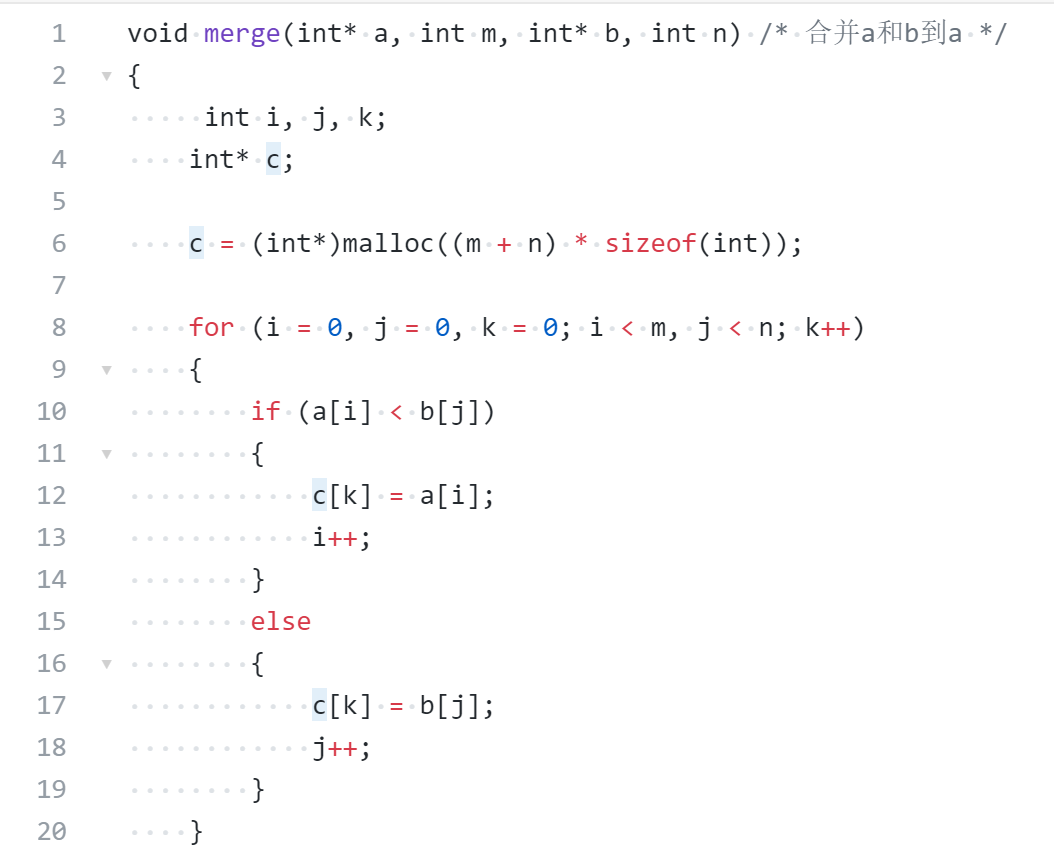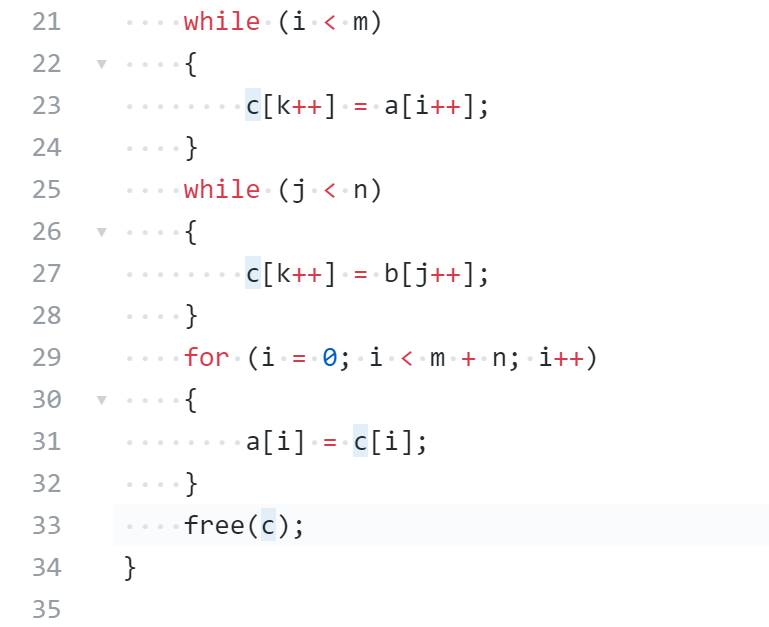### 2.2.3 找一份同学代码（尽量找思路和自己差距较大同学代码）比较，说明各自代码特点。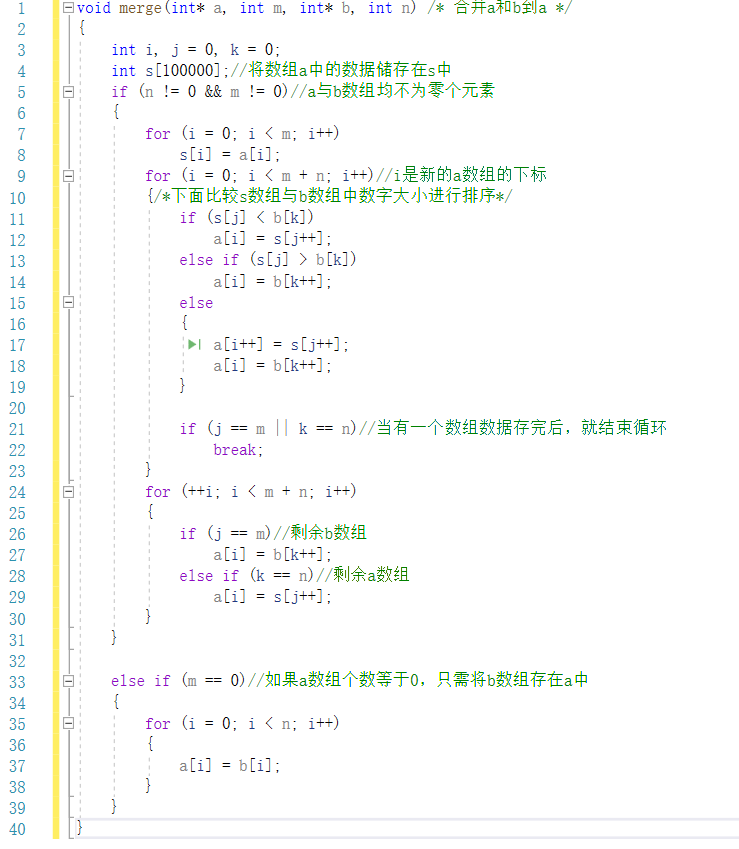## 2.3 说反话-加强版（3分）

### 2.3.1 伪代码

for i=0 to len
if(s[i]!=' ')
把此时的i储存在min中
break;
end if
end for
for i=len-1 to 0 , i--
if (s[i] == ' ' && ((s[i + 1] >= 'a' && s[i + 1] <= 'z') || (s[i + 1] >= 'A' && s[i + 1] <= 'Z')))//找到最后一个单词的首字符
for  j=i+1 to p , j++
if (s[j] == ' ')
break;
end if
输出字符串s[j];
end for
if((j-1)!=min)//防止最后一个单词多输出空格
输出空格
end if
p=i;
end if
end for
for i=0 to min+1 do i++
输出字符串s[i];
end for


### 2.3.2 代码截图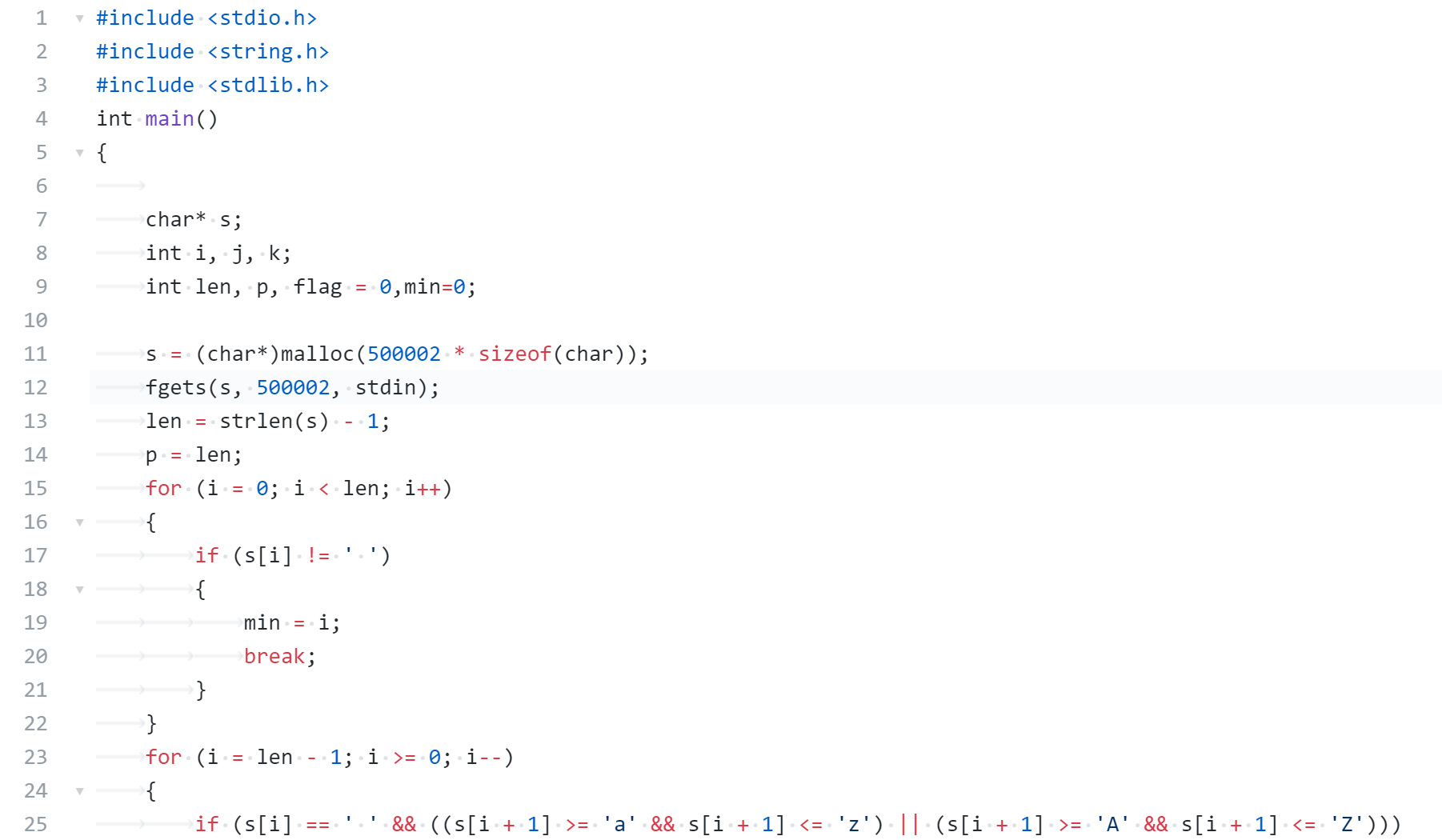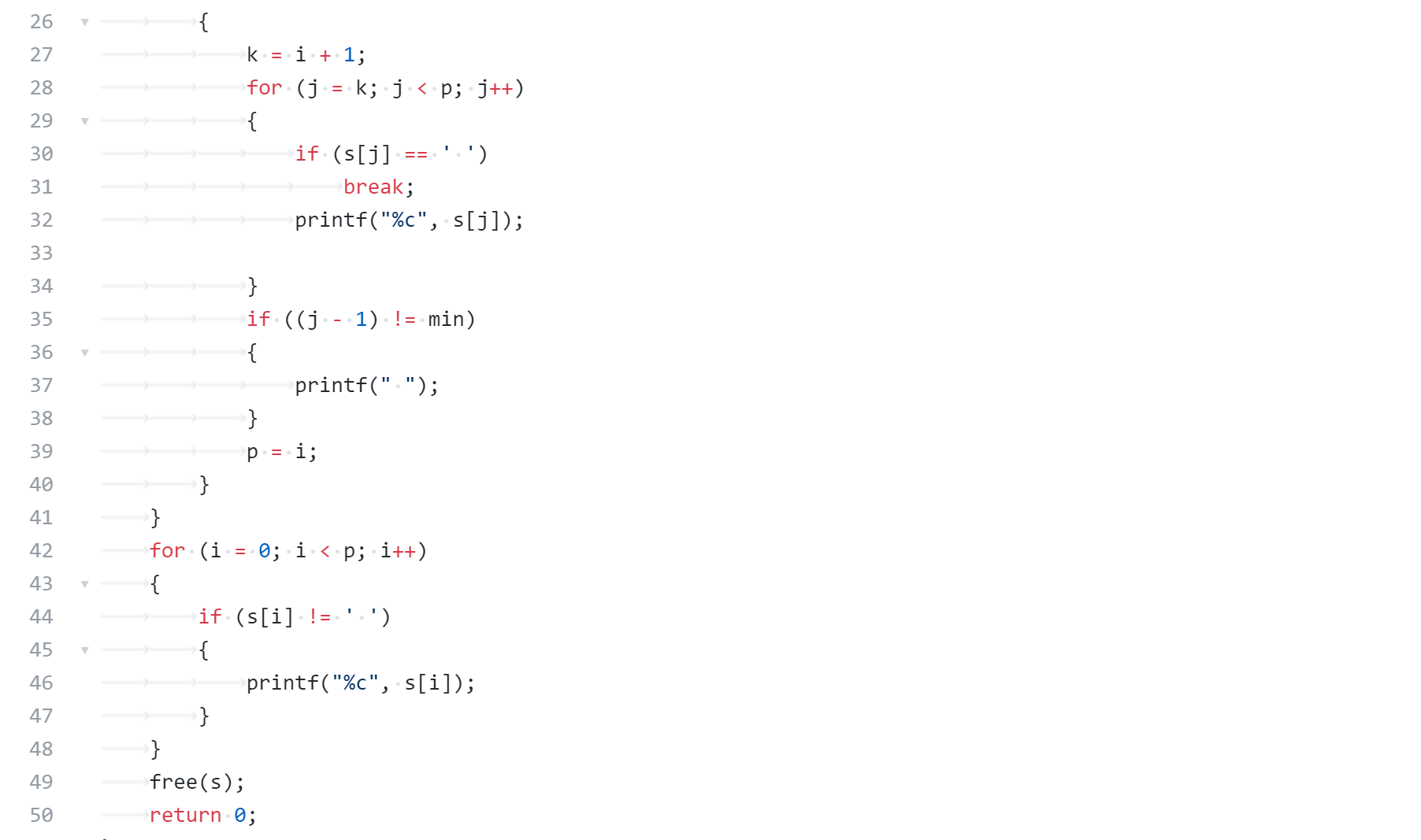### 2.3.3 请说明和超星视频做法区别，各自优缺点。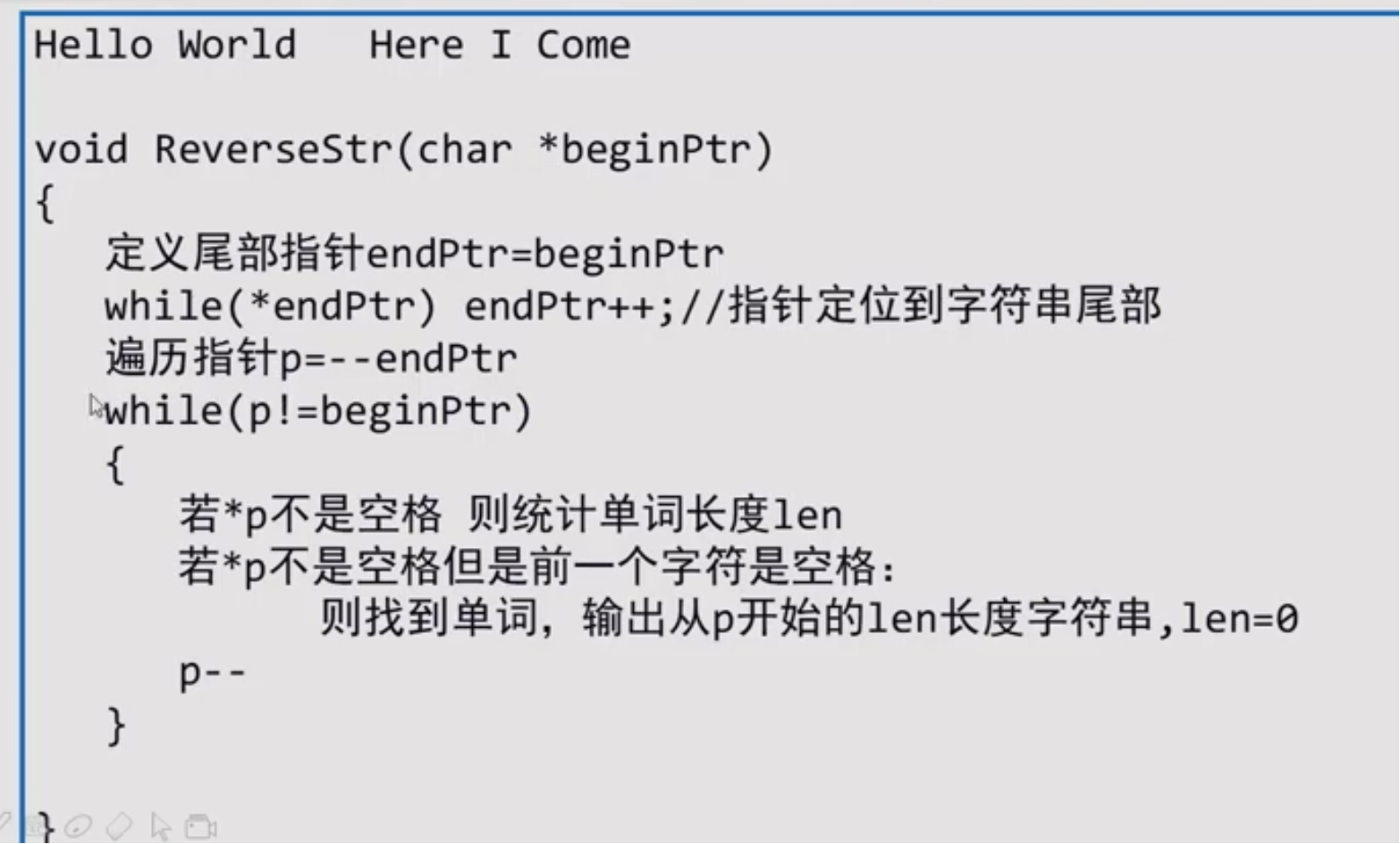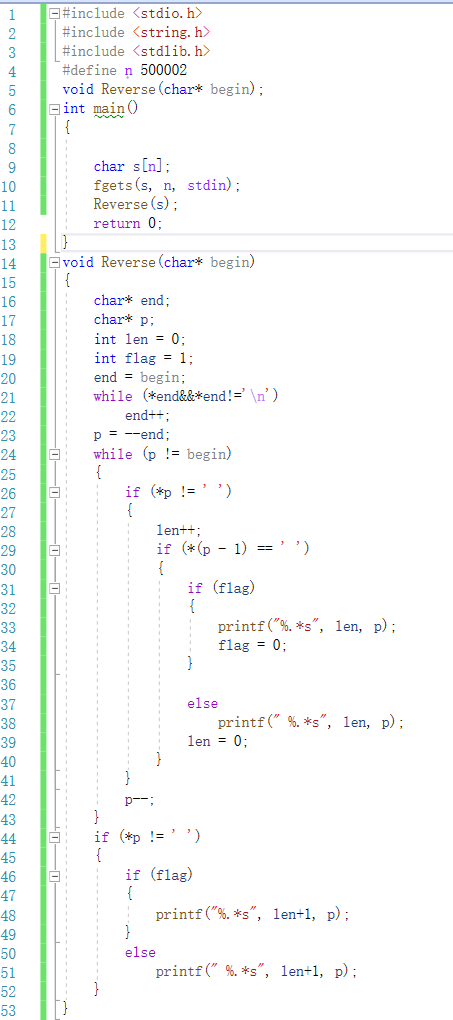posted @ 2020-12-27 19:52  SmileCHT  阅读(94)  评论(0编辑  收藏  举报